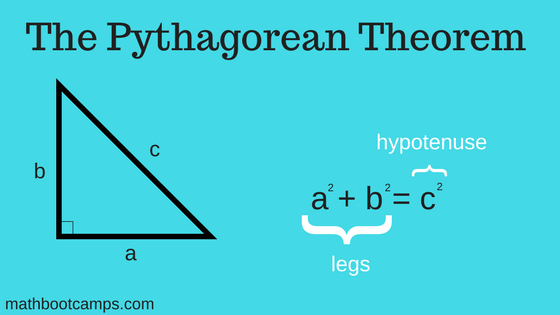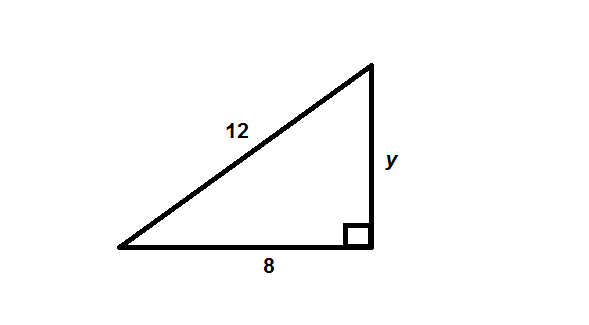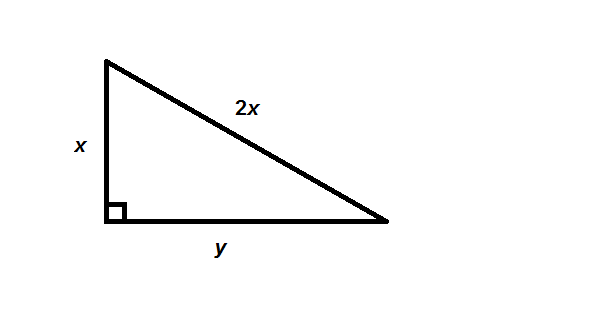# The Pythagorean theorem with examples

The Pythagorean theorem is a way of relating the leg lengths of a right triangle to the length of the hypotenuse, which is the side opposite the right angle. Even though it is written in these terms, it can be used to find any of the side as long as you know the lengths of the other two sides. In this lesson, we will look at several different types of examples of applying this theorem.

## Applying the Pythagorean theorem (examples)

In the examples below, we will see how to apply this rule to find any side of a right triangle triangle. As in the formula below, we will let a and b be the lengths of the legs and c be the length of the hypotenuse. Remember though, that you could use any variables to represent these lengths.In each example, pay close attention to the information given and what we are trying to find. This helps you determine the correct values to use in the different parts of the formula.

### Example

Find the value of $$x$$.### Solution

The side opposite the right angle is the side labelled $$x$$. This is the hypotenuse. When applying the Pythagorean theorem, this squared is equal to the sum of the other two sides squared. Mathematically, this means:

$$6^2 + 8^2 = x^2$$

Which is the same as:

$$100 = x^2$$

Therefore, we can write:

\begin{align}x &= \sqrt{100}\\ &= \bbox[border: 1px solid black; padding: 2px]{10}\end{align}

Maybe you remember that in an equation like this, $$x$$ could also be –10, since –10 squared is also 100. But, the length of any side of a triangle can never be negative and therefore we only consider the positive square root.

In other situations, you will be trying to find the length of one of the legs of a right triangle. You can still use the Pythagorean theorem in these types of problems, but you will need to be careful about the order you use the values in the formula.

### Example

Find the value of $$y$$.### Solution

The side opposite the right angle has a length of 12. Therefore, we will write:

$$8^2 + y^2 = 12^2$$

This is the same as:

$$64 + y^2 = 144$$

Subtracting 64 from both sides:

$$y^2 = 80$$

Therefore:

\begin{align}y &= \sqrt{80} \\ &= \sqrt{16 \times 5} \\ &= \bbox[border: 1px solid black; padding: 2px]{4\sqrt{5}}\end{align}

In this last example, we left the answer in exact form instead of finding a decimal approximation. This is common unless you are working on an applied problem.

## Applications (word problems) with the Pythagorean theorem

There are many different kinds of real-life problems that can be solved using the Pythagorean theorem. The easiest way to see that you should be applying this theorem is by drawing a picture of whatever situation is described.

### Example

Two hikers leave a cabin at the same time, one heading due south and the other headed due west. After one hour, the hiker walking south has covered 2.8 miles and the hiker walking west has covered 3.1 miles. At that moment, what is the shortest distance between the two hikers?

### Solution

First, sketch a picture of the information given. Label any unknown value with a variable name, like x.Due south and due west form a right angle, and the shortest distance between any two points is a straight line. Therefore, we can apply the Pythagorean theorem and write:

$$3.1^2 + 2.8^2 = x^2$$

Here, you will need to use a calculator to simplify the left-hand side:

$$17.45 = x^2$$

Now use your calculator to take the square root. You will likely need to round your answer.

\begin{align}x &= \sqrt{17.45} \\ &\approx 4.18 \text{ miles}\end{align}

As you can see, it will be up to you to determine that a right angle is part of the situation given in the word problem. If it isn’t, then you can’t use the Pythagorean theorem.

## Algebra style problems with the Pythagorean theorem

There is one last type of problem you might run into where you use the Pythagorean theorem to write some type of algebraic expression. This is something that you will not need to do in every course, but it does come up.

### Example

A right triangle has a hypotenuse of length $$2x$$, a leg of length $$x$$, and a leg of length y. Write an expression that shows the value of $$y$$ in terms of $$x$$.

### Solution

Since no figure was given, your first step should be to draw one. The order of the legs isn’t important, but remember that the hypotenuse is opposite the right angle.Now you can apply the Pythagorean theorem to write:

$$x^2 + y^2 = (2x)^2$$

Squaring the right-hand side:

$$x^2 + y^2 = 4x^2$$

When the problem says “the value of $$y$$”, it means you must solve for $$y$$. Therefore, we will write:

$$y^2 = 4x^2 – x^2$$

Combining like terms:

$$y^2 = 3x^2$$

Now, use the square root to write:

$$y = \sqrt{3x^2}$$

Finally, this simplifies to give us the expression we are looking for:

$$y = \bbox[border: 1px solid black; padding: 2px]{x\sqrt{3x}}$$